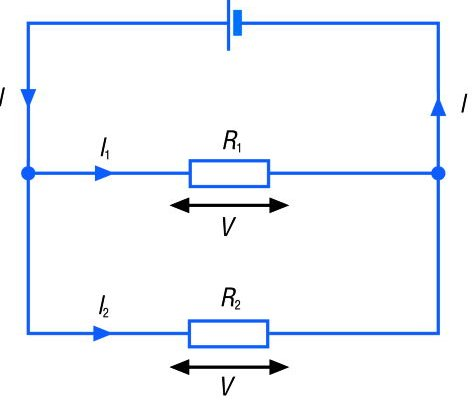A# parallel circuitA parallel circuit is an electrical circuit in which the components are connected side by side. The current flowing in the circuit is shared by the components.

## Resistance in a parallel circuit

The total resistance RT of two conductors of resistances R1 and R2 connected in parallel is given by:

1/RT = 1/R1 + 1/R2

## Voltage in a parallel circuit

Components are connected across the same voltage source, and therefore the voltage across each of the components is the same. This fact can be expressed mathematically as VT = V1 = V2, where VT is the total voltage, V1 is the voltage across component 1, and V2 is the voltage across component 2. Appliances are connected in parallel circuits with the same voltage applied to each appliance.

## Current in a parallel circuit

The current divides to flow through each of the components in the parallel paths. The amount of current that flows through each of the components depends on the resistance of each component. A component with a lower resistance will allow more current to flow through. If I1 is the current flowing through component 1 and I2 is the current flowing through component 2, then the total current, IT, is given by IT = I1 + I2.